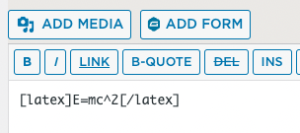'

# Displaying Mathematical Equations Demo

## A Simple Example

Detailed documentation can be found at https://docs.mathjax.org/en/latest/

Surround the notation, in html/Text view, with the WordPress Shortcode tag “latex” (rather than the Javascript delimiter, ) as in this screenshot:The result is as follows:
$$E=mc^2$$

## A Longer Example

Surround x = {-b \pm \sqrt{b^2-4ac} \over 2a} with the latex shortcode and you get the following:

$$x = {-b \pm \sqrt{b^2-4ac} \over 2a}$$